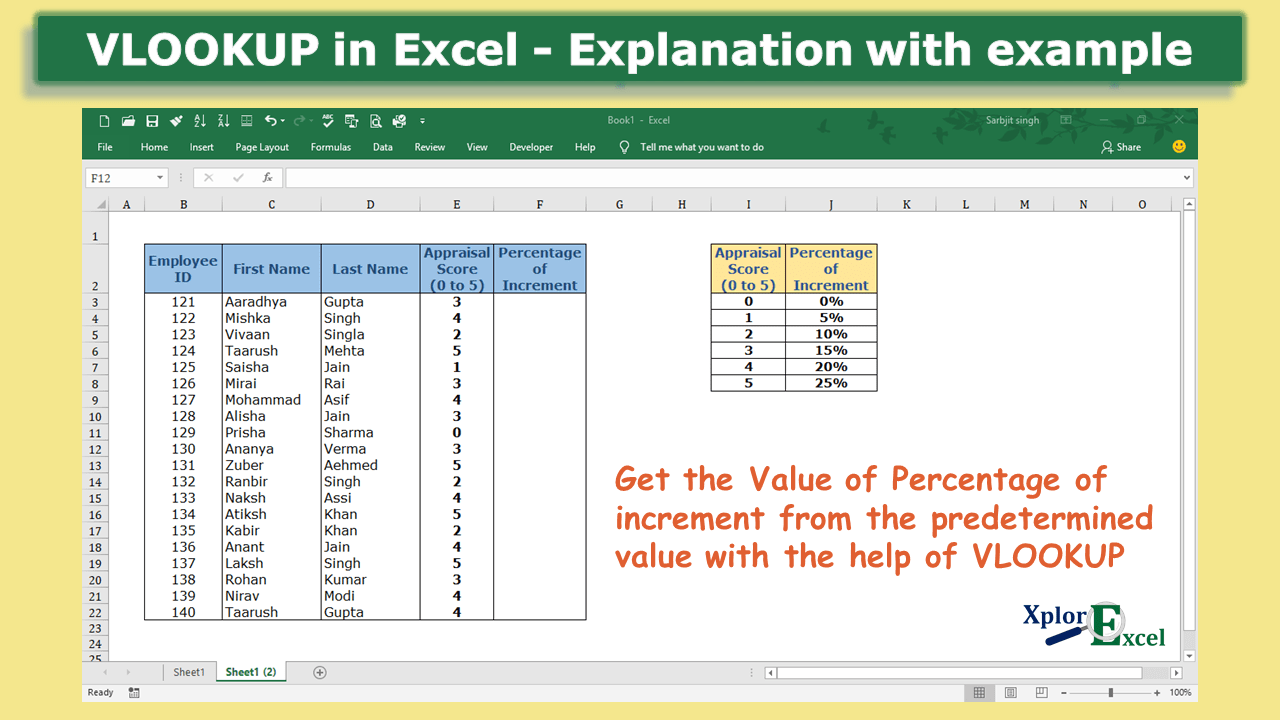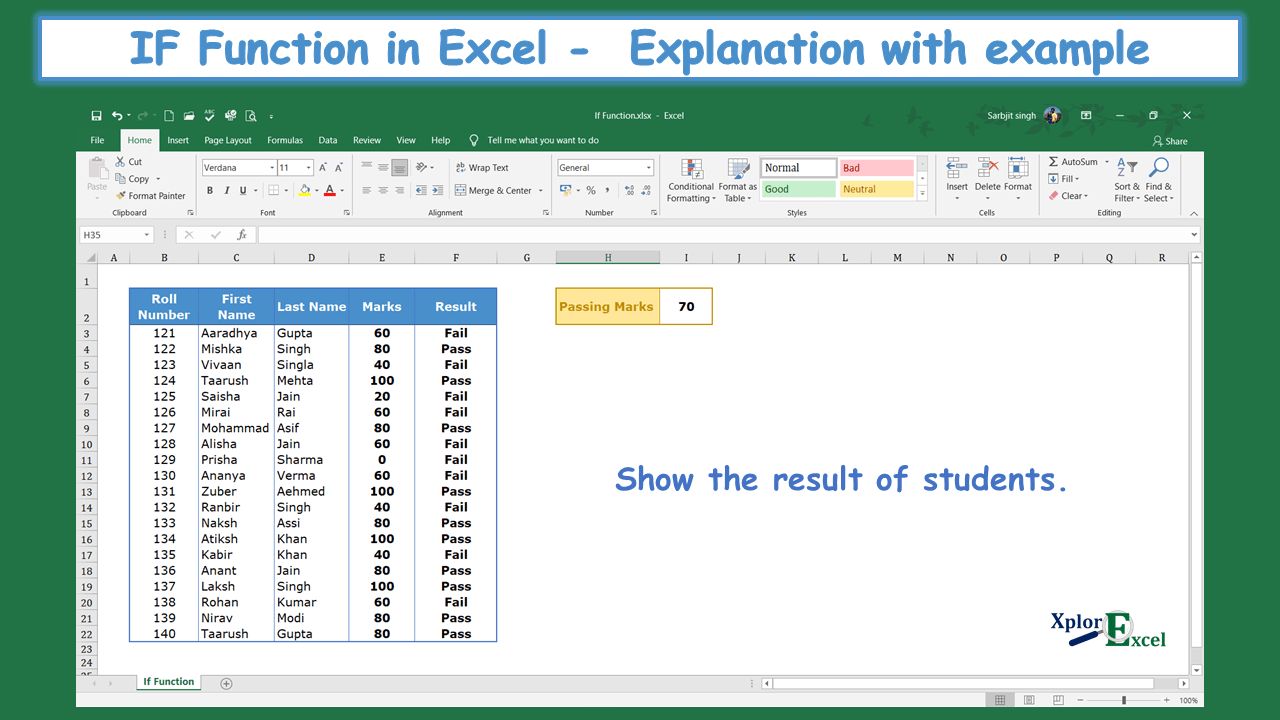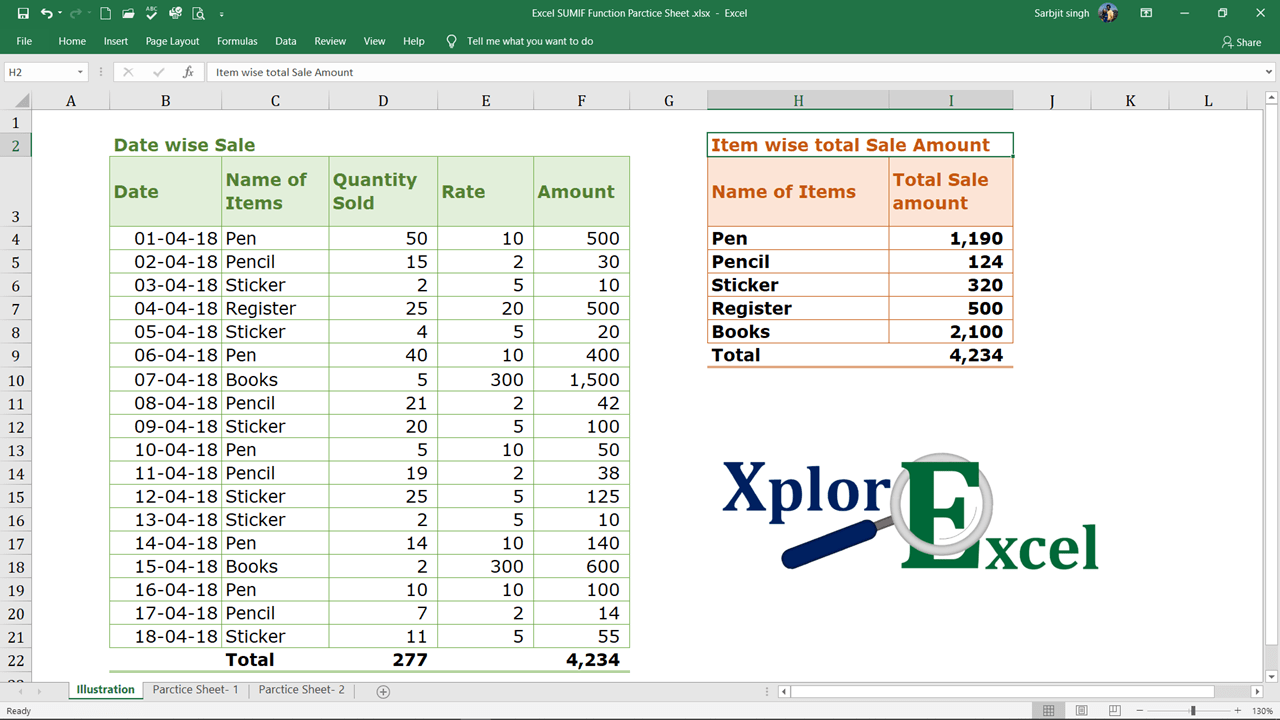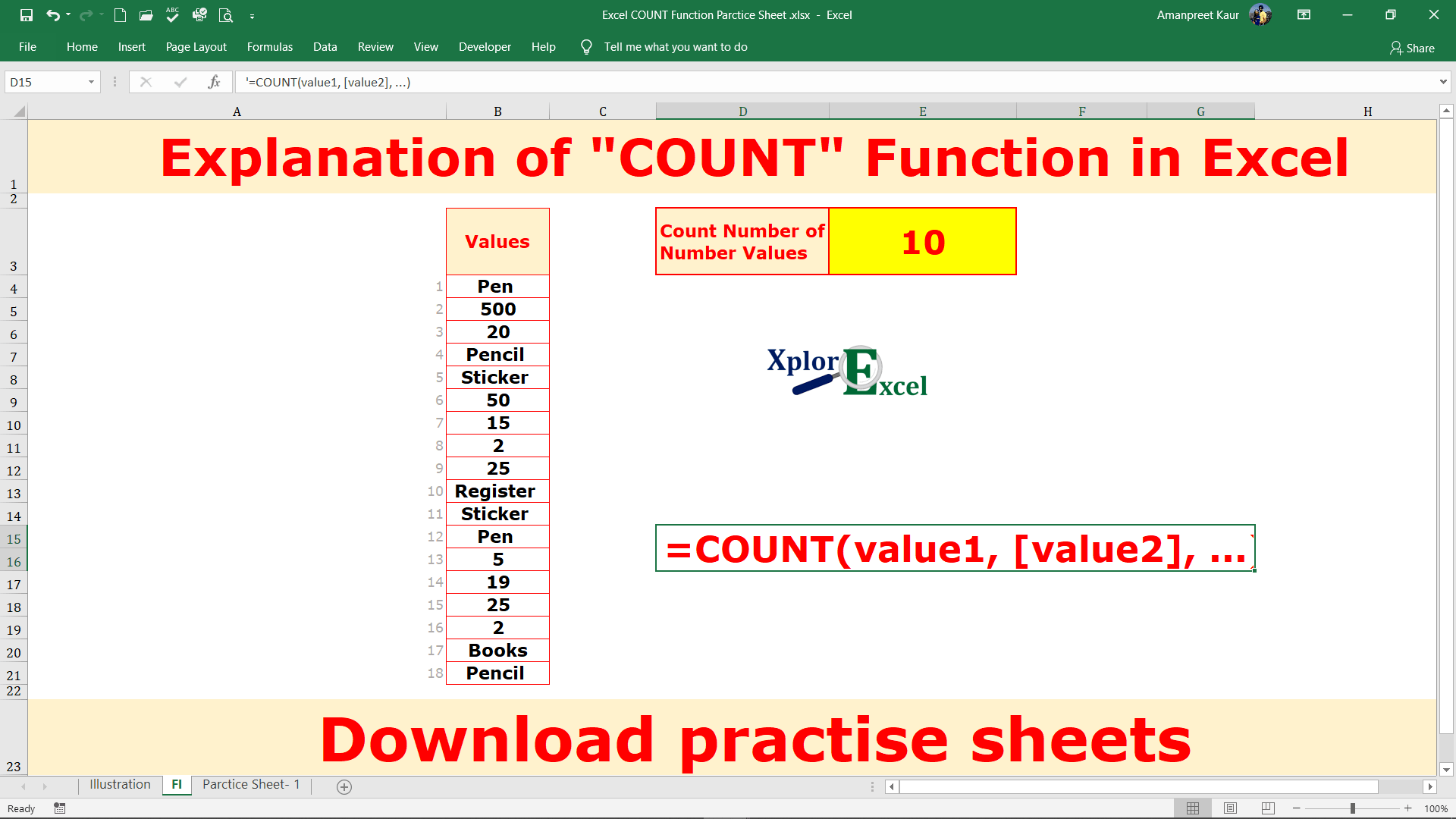Microsoft Excel has so much potential to do the daily repeated tasks within seconds which take more than 1 minute, 1 hour, 1 day, etc. The Excel Formulas and Excel Shortcuts will reduce your working hour and increase your efficiency.

## Microsoft Excel Formulas: –

There are a number of formulas in Microsoft Excel that will simplify your day-to-day work and increase your speed of working. you can do your repeated or daily task with just one click or automatic. You just have to put data into you workbook rest of all your repeated task will perform by excel itself.### How to use VLOOKUP in Excel – free explanation

What is VLOOKUP: - In VLOOKUP, V stands for vertical and with the help of this formula, we can retrieve the ...### How to use HLOOKUP in Excel -Free Explanation

HLOOKUP in Excel: In the HLOOKUP Function "H" stand for horizontal and with the help of this formula, we can ...### IF Function in Excel – Free Explanation with example

IF Function in Excel: - The "IF" Function in Excel is a logical test, representing the result in two ways ..."SUMIF" function is used to know the total of the particular item from the given data. What is the "SUMIF" ...Excel Match Function:- The Excel Match function is used to determine the selected value position in the selected array. The ...Excel Index Function:- The Excel Index function is used to find out the value in a selected array or range by ...